NEET  >  Sample Test: Physics

# Sample Test: Physics

Test Description

## 20 Questions MCQ Test NEET Mock Test Series | Sample Test: Physics

Sample Test: Physics for NEET 2023 is part of NEET Mock Test Series preparation. The Sample Test: Physics questions and answers have been prepared according to the NEET exam syllabus.The Sample Test: Physics MCQs are made for NEET 2023 Exam. Find important definitions, questions, notes, meanings, examples, exercises, MCQs and online tests for Sample Test: Physics below.
Solutions of Sample Test: Physics questions in English are available as part of our NEET Mock Test Series for NEET & Sample Test: Physics solutions in Hindi for NEET Mock Test Series course. Download more important topics, notes, lectures and mock test series for NEET Exam by signing up for free. Attempt Sample Test: Physics | 20 questions in 20 minutes | Mock test for NEET preparation | Free important questions MCQ to study NEET Mock Test Series for NEET Exam | Download free PDF with solutions
 1 Crore+ students have signed up on EduRev. Have you?
Sample Test: Physics - Question 1

### In an n-p-n transistor, 108 electrons are emitted from the emitter in 10-4 s. If  2% of electrons are lost in the base. Then the current gain in common-emitter configuration mode is

Detailed Solution for Sample Test: Physics - Question 1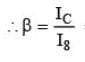= (98/100) / (2/100)
= 98/2
= 49

Sample Test: Physics - Question 2

### Electromagnetic radiations of intensity I is incident normally on a perfectly reflecting surface. If speed of light is C, then radiation pressure exerted on the surface is

Detailed Solution for Sample Test: Physics - Question 2

Let no. of photons falling per second per unit area = n
So, I = nhf
Pressure = Force / Area = 2n(h / λ) = 2nhf / c = 2I / C

Sample Test: Physics - Question 3

### A simple microscope has a focal length of 2.5 cm. Maximum angular magnification of it will be (Given that least distance of clear vision = D = 25 cm)

Detailed Solution for Sample Test: Physics - Question 3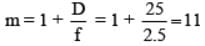Sample Test: Physics - Question 4

Suppose θ is polarising angle for a transparent medium and speed of light in that medium is v, then according to Brewster law.

Detailed Solution for Sample Test: Physics - Question 4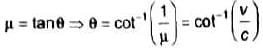Sample Test: Physics - Question 5

A convex lens made up of glass (μ = 1.5) has 20 cm focal length in air (μ = 1). Then its focal length in water  (μ = 1.33) will be:

Detailed Solution for Sample Test: Physics - Question 5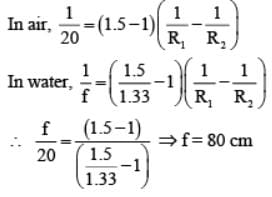Sample Test: Physics - Question 6

An eye specialist prescribes spectacles having a combination of a convex lens of focal length 40 cm in contact with a concave lens of focal length 25 cm. The power of this lens combination is

Detailed Solution for Sample Test: Physics - Question 6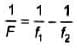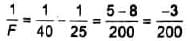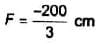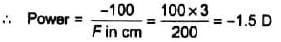Sample Test: Physics - Question 7

A nucleus of mass number 220, initially at rest emits an α -particle. If the Q value of the reaction is 5 MeV, then the energy of emitted α -particle will be

Detailed Solution for Sample Test: Physics - Question 7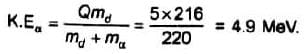Sample Test: Physics - Question 8

A sample of radioactive material has a mass m, decay constant λ and molecular weight M. If avogadro’s constant is NA. Then initial activity of the sample is

Detailed Solution for Sample Test: Physics - Question 8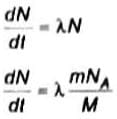Sample Test: Physics - Question 9

Two nucleons are at a separation of 1 fm. The net force between them is F1 if both are neutrons, F2 if both are protons, and F3 if one is a proton and the other is a neutron.

Detailed Solution for Sample Test: Physics - Question 9

Nuclear forces will be same all three cases but total force F2 will be smaller because of electrostatic repulsion between two protons.

Sample Test: Physics - Question 10

Suppose the potential energy between electron and proton at a distance r is vary as U ∝ r2. Assuming Bohr's model of quantization of angular momentum and circular orbits, radius of the nth allowed orbit is proportional to

Detailed Solution for Sample Test: Physics - Question 10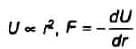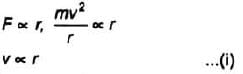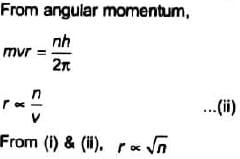Sample Test: Physics - Question 11

The ionization potential of hydrogen atom is − 13.6 V. Photons of energy 12.75 eV are made to incident on sample of H atoms at ground state. How many spectral lines are expected in emitted radiation?

Detailed Solution for Sample Test: Physics - Question 11

E=E1/n2
Energy used for excitation is 12.75 eV
ie, (-13.6+12.75)eV = -0.85eV image
Energy levels of H-atom
The photons of energy 12.75eV can excite the fourth level of H-atom. Therefore, six lines will be emitted. [ n (n−1) /2 lines].

Sample Test: Physics - Question 12

de-Brogile wavelength associated with a proton accelerated from rest by 10 kV is λ. The de-Brogile wavelength associated with a 10 keV neutron is

Detailed Solution for Sample Test: Physics - Question 12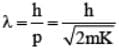, where k is kinetic energy of particle. According to given data, kinetic energy of proton is 10keV which is same as that of neutron. Masses are also same.

Sample Test: Physics - Question 13

A body of mass 6 kg is lowered down with help of a string with downward acceleration g/4. The work done by the string on the body in first 3s after start of the motion is (g = 10 m/s2)

Detailed Solution for Sample Test: Physics - Question 13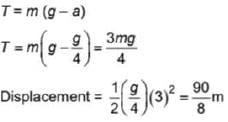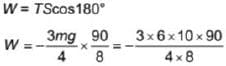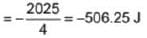Sample Test: Physics - Question 14

Light from three non-coherent sources, having same intensity, interfere at a location and produce resultant intensity 6I. If one of the three sources is switched off, then the resultant intensity at same point will be

Detailed Solution for Sample Test: Physics - Question 14

In case of non-coherent sources I net = ∑l
Let intensity from one source by l
⇒ 3lo = 6l
⇒ lo = 2I
If one source is switched off
Net intensity = 2Io = 4I

Sample Test: Physics - Question 15

Figure shows a flywheel of radius 10 cm. Its moment of inertia about the axis of rotation is 0.4 kg m2. A massless string passes over the flywheel and a mass of 2 kg attached at its lower end. Angular acceleration of flywheel in (rad/s2) is nearly (g = m/s2)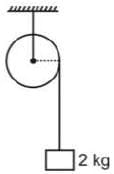Detailed Solution for Sample Test: Physics - Question 15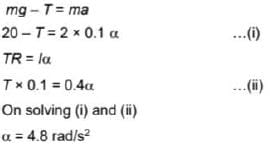Sample Test: Physics - Question 16

Radius of a planet is three times the radius of the earth and it has same average density as that of the earth. The ratio of escape speeds from surface of the planet and that from the surface of earth is

Detailed Solution for Sample Test: Physics - Question 16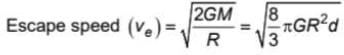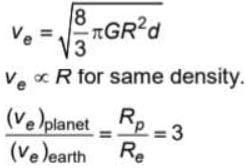Sample Test: Physics - Question 17

Heat is supplied to a certain sample of a substance in solid state at a constant rate. The variation of temperature is plotted against time as shown in the figure. Choose the correct statement regarding the sample.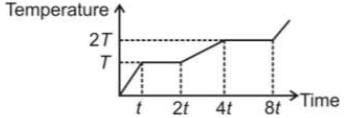Detailed Solution for Sample Test: Physics - Question 17

Since time required for same temperature increase is larger in liquid state than solid state, the specific heat capacity is higher in liquid state. Since in phase transition, the heat required is less in fusion than evaporation, hence latent heat of fusion is less.

Sample Test: Physics - Question 18

A simple pendulum has a time period T in vacuum. Its time period when it is completely immersed in a nonviscous liquid of density one-fourth density of material of bob is

Detailed Solution for Sample Test: Physics - Question 18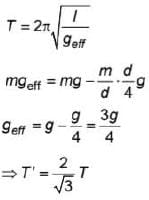Sample Test: Physics - Question 19

A car is moving on a road with a speed 40 m/s, blows a horn of frequency 200 Hz. The apparent wavelength of sound as observed by a detector on the road behind the car will be (Speed of sound in air is 340 m/s)

Detailed Solution for Sample Test: Physics - Question 19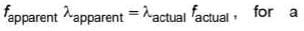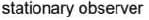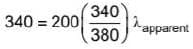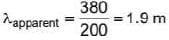Sample Test: Physics - Question 20

The resistance of a galvanometer coil is 48 Ω . The resistance of shunt required to convert it into an ammeter of range 6 times as that of galvanometer is

Detailed Solution for Sample Test: Physics - Question 20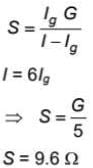## NEET Mock Test Series

1 videos|25 docs|144 tests
 Use Code STAYHOME200 and get INR 200 additional OFF Use Coupon Code
Information about Sample Test: Physics Page
In this test you can find the Exam questions for Sample Test: Physics solved & explained in the simplest way possible. Besides giving Questions and answers for Sample Test: Physics , EduRev gives you an ample number of Online tests for practice

## NEET Mock Test Series

1 videos|25 docs|144 tests

### How to Prepare for NEET

Read our guide to prepare for NEET which is created by Toppers & the best Teachers